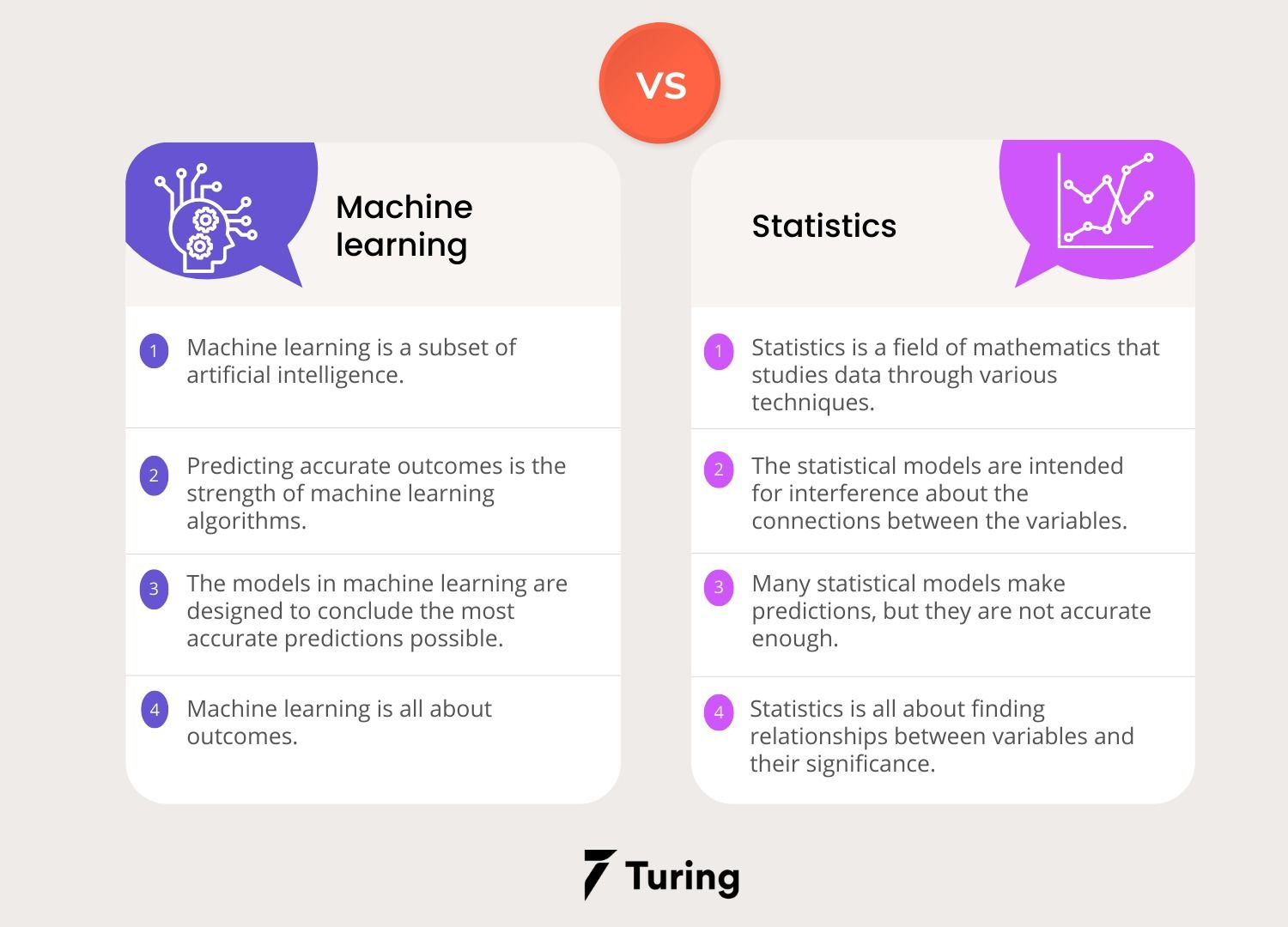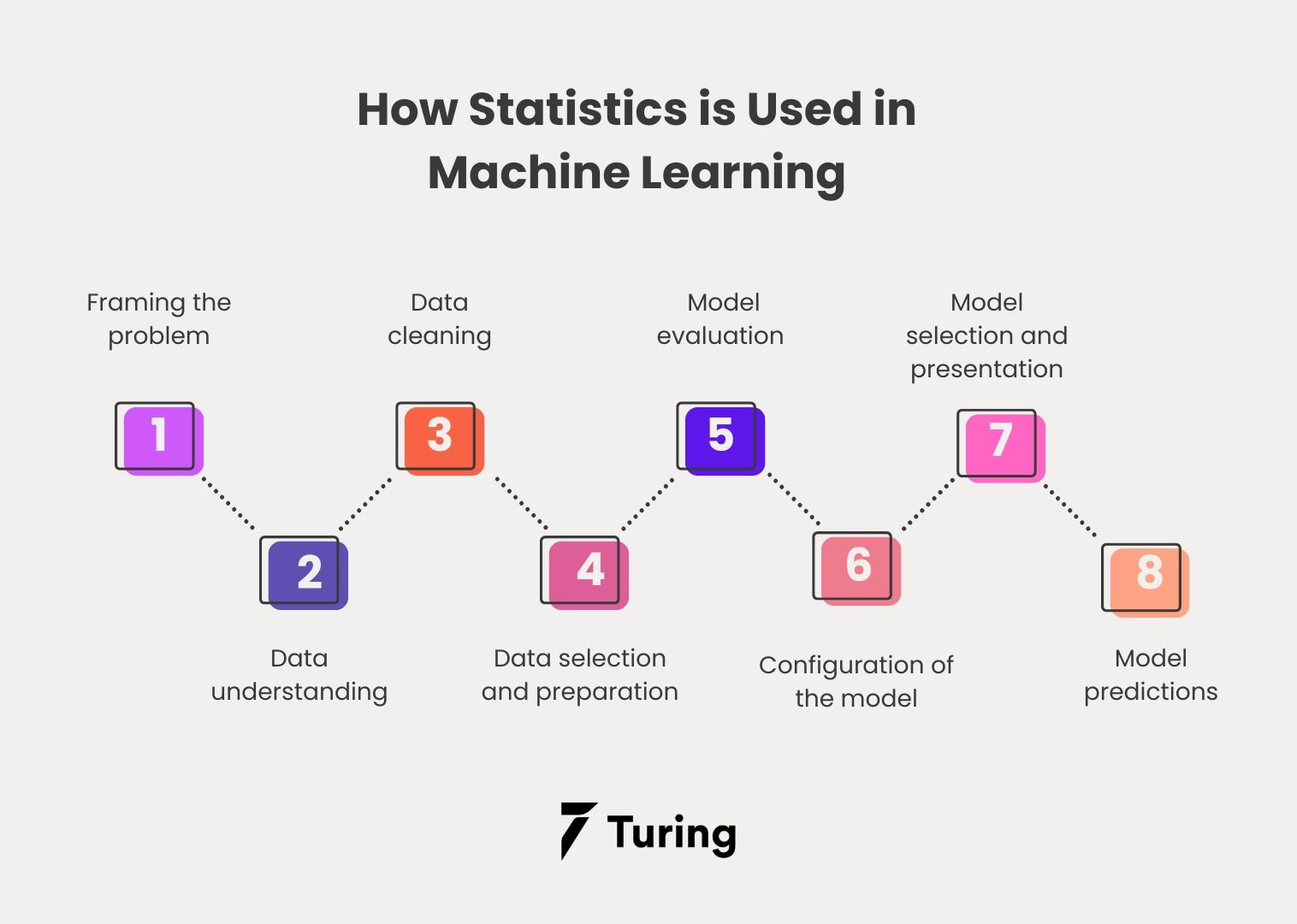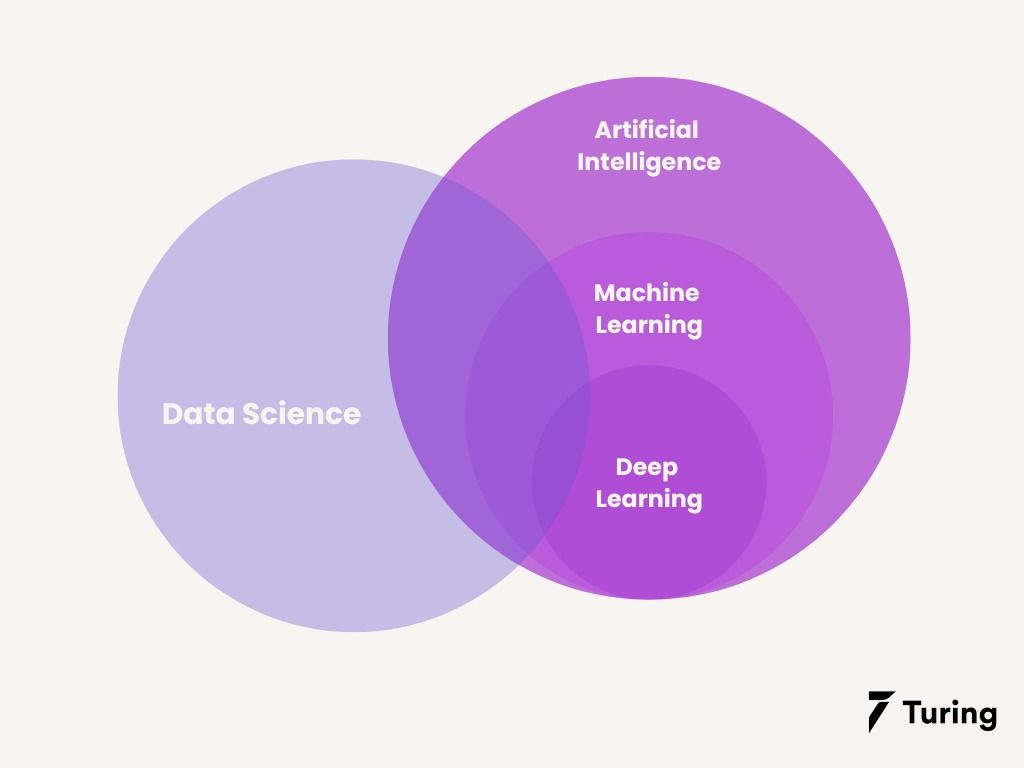# Introduction to Statistics for Machine LearningMachine learning is a subset of artificial intelligence in which a model holds the capability of automatically learning from the data over a period. The algorithm uses these teachings for model predictions. These predictions of the machine learning algorithm become more accurate as we feed more data to it.

Statistics for machine learning come as a significant tool that studies this data for recognizing certain patterns. It helps you find unseen patterns by providing a proper direction for utilizing, analyzing, and presenting the raw data that is successfully implemented in fields like computer vision and speech analysis.

Let's get started with understanding why we need statistics for machine learning.

## Statistics for machine learning

The statistics for machine learning comealong in the first phase of machine learning algorithm. It helps us to deal with the data, as it is the foundation of implementing statistical concepts and ultimately interpreting conclusions from it.

To simplify, it is a mathematics field used for collecting, organizing, and analyzing data.

However, to understand the work of statistics under the hood, we need to have knowledge about its subcategories. These are as follows:

### Descriptive statistics

This consist of organizing and summarizing the data with the help of graphs and numbers. For instance: histograms, pie charts, graphs, and others. We can perform it on population data and on a sample.

### Inferential statistics

This uses data to reach conclusions. Various tests are performed on the sample data, including data visualization, manipulation, and others to conclude different decisions.

Now, with a basic understanding of the basic statistics concepts, if we lay down the point of differences between statistics and machine learning, the picture would look as given below.

## A brief on machine learning v/s statistics

Several vague statements gave birth to the myth that machine learning and statistics are synonymous. But, they are not the same. There is an enormous difference between statistics and machine learning that we will bring to light in the next segment.With all said above, stating that machine learning is based on statistical learning theory, things would make sense to you. To make things clearer, we will shed more light on the relationship between statistics and machine learning.

## Relation between statistics and machine learning

If we talk about the relationship between statistics and machine learning, ‌their principal goals are different, but they are closely related in terms of the methods used in each. In machine learning, the focus is on the results and model skill instead of factors like model interpretability. Statistics focus more on the explainability of the predictions made and the behavior of models.

So, when we talk about machine learning and statistics as distinct fields, both offer different perspectives on the same problem. They are like the two wheels of a bicycle that can’t work without one another. The practitioners of both fields need to monitor each other ‌to deliver real and valuable contributions to the problems.

For a clear understanding of the relationship between both the domains, we have added some more points below:

• Both fields leverage observation that can be one or an entire vector of attributes.
• Both fields predict or estimate the output based on the input fed.
• In statistics, maximizing the likelihood of predicting the model parameters is equivalent to minimizing the entropy to derive the best parameters in machine learning.
• A hypothesis in statistics and the prediction rule in machine rule are similar and each needs to be scrutinized.
• Both fields translate data into quantitative claims for accurate outcomes when the supply of relevant data increases.

This sums up the relationship between machine learning and statistics and how they are similar in so many aspects.

Now, to understand the working of each, we need to understand them separately and then together. Let’s get started by understanding the basic statistic concepts with an example.

## Basics of statistics for machine learning

Suppose you want to know what is the most common height of students in your class. You can either calculate the mean or mode or median, right? This is exactly what we call descriptive statistics. We are trying to summarize the data with the help of any statistical method.

Now if you want to know, the height of the students in this classroom similar to what you expect in a normal class at this university? You might say that yes, the heights of the students in the class are very similar to that of the other students in the university. Hence, here you are trying to get some conclusions from your data.

There are various types of tests to conclude the result from your data. Some of them are z-test, t-test, chi-square test, ANOVA, etc. We will learn about all the tests in the upcoming article. In this article, we will first build up our base and then we will move ahead.

### Important terminologies

Let’s understand a few important terms that are required to understand machine learning statistics and are repeated over and over in this article.

Population (N): Suppose you want to know the average age of the people in India. Is it possible? Can you reach out to every single person and ask their age? Definitely not. In this case, the count of people living in India makes up for our population. The population is mostly denoted by capital ‘N’.

Sample (n): To know the average age of people living in India, a better approach will be to take out a few samples from this population and study them. We can definitely not reach 130cr people but we can surely reach out to a few people and ask them their age. This process is called sampling. The population here is mostly denoted by small ‘n’.

Variable: A variable is any characteristic, number, or quantity that can be measured or counted, or we can even say that variable is a property that can take on many values. For example, ‘income’ is a variable that can vary between data units in a population or it can also vary over time for each data unit. Let’s see various types of variables:

- Quantitative variables- these variables are measured numerically, and that is the reason we can add, subtract, multiply, or divide to get a meaningful result. For example, “age”, “no of students in a class”. Quantitative variables are further classified into 2 more types:

• Discrete variable: It is the one whose value is calculated by counting. For example, counting the number of girls in the class.

• Continuous variable: It is the variable that represents measurable amounts like weight, volume, or others.

- Qualitative/categorical variable- these variables can take on names and labels and can fit into categories. For example “gender” can have two labels ‘male’ and ‘female’, another example might be “breed of a dog” which can have multiple types like ‘bulldog’, ‘poodle’, ‘lab’ etc. Most of the machine learning problem statements you’ll get will have their output as a qualitative variable.

Now you must be wondering, on what basis do we take samples from this huge population? Well, statistics have it all covered. There are various types of sampling methods ‌we use to meet our requirements. Read them below as we have discussed each one of them in brief.

### Sampling techniques in Statistics

1. Simple random sampling - As the name suggests, the samples are randomly selected. This means each person here in our case has an equal probability of getting selected in the case of simple random sampling.

2. Stratified sampling - If we divide this population into smaller groups, we can use the stratified sampling method. Another term for groups is “strata”, you may find it in books or other articles. Improved quality of data may be obtained by employing different types of people in different strata/groups. For example, people knowing local languages may be deployed to the rural areas, whereas in urban areas people knowing english may be more advantageous. Or we can even divide this population into males and females.

3. Systematic sampling - This is a probability sampling method where we randomly choose a starting point, let's say (n) and thereafter at a certain interval the next element is chosen for the sample. Let’s say you choose a starting point as 4 which means you will ask every 4th person about their age as you move ahead. But this has certain disadvantages. Mainly if you are near an old age home and you decide to ask the age of every 4th person you meet. You will probably end up getting 60+ age and that will be extremely biased since you started taking samples near an old age home.

4. Convenience sampling - It is also called voluntary response sampling. Suppose you are taking a survey and you have mailed it to 2000 people. Will everyone fill the survey form? Obviously, the answer is no. Only the people who are interested in this survey will fill up the form. Hence in convenience sampling we basically pick people who are interested in sharing their age (for our example).

## Use of statistics in machine learningThe uses of statistics in machine learning are incredible. We have elaborated each one of them below.

1. Framing the problem

Problem framing is a prominent point under statistics in machine learning use cases.

This aspect in a predictive modelling problem requires significant exploration of the observations in the domain for newcomers. However, for the domain experts, it helps in considering the data from multiple perspectives.

The statistical method that helps in data exploration while framing a problem include data mining and exploratory data analysis.

2. Data understanding

Data understanding refers to grasping the relationship between variables and their distribution. We leverage two major branches of statistical methods in understanding data in an applied machine learning project. These are summary statistics and data visualisation.

3. Data cleaning

It refers to the process of identifying and repairing issues related to the data. Even though all the data is digital, it can sometimes disrupt the models or processes in cases like data loss, errors, and corruption.

Imputation and outlier detection are the two statistical methods we use for data cleaning in a machine learning project.

4. Date selection and preparation

Not every variable or observation is relevant while modeling. The process of data selection is where we reduce the data to make it relevant for predictions. We leverage two types of statistical machine learning methods for data selection, namely feature selection and data sample.

Once the relevant data is on the table, it is transformed to change its structure or shape. It is done to make it more suitable for the chosen framing of the learning algorithms. This process of transforming the data to be used for modeling is referred to as data preparation. It is performed using three statistical methods namely scaling, encoding, and transforms.

5. Model evaluation

Evaluating the learning method is a crucial part of predictive modeling problems. There’s an entire subfield of statistical methods called experimental design that plans the process of training and evaluating a predictive model.

Further, while implementing the experimental design, there are methods used for making economical use of ‌data in order to predict the model skill. It is referred to as the resampling method in which the dataset is systematically split into subsets for training and evaluation purposes in a predictive model.

6. Configuration of the model

The learning methods included in the suite of hyper-parameters in a ‌machine learning algorithm are flexible to be tailored as per a given problem. Thus, one of the two subfields of statistics used in machine learning model, namely statistical hypothesis tests and estimation statistics is leveraged for interpretation and comparison of the results between different hyperparameter configurations.

7. Model selection and presentation

Model selection refers to the process of selecting one method or machine learning algorithm among many as the solution for a given predictive modeling problem. Two classes of statistical methods come in handy to interpret the estimated skill required for the purpose of model selection. These are statistical hypothesis tests and estimation statistics.

Once the training of the final model is completed, it is presented before the stakeholders to showcase the estimated skill of the model. This process is known as model presentation.

It is done prior to being deployed or used to make actual predictions on real data. We leverage estimation statistics as a method to quantify the uncertainty involved in the estimated skill of a particular machine learning model through the use of confidence and tolerance intervals.

8. Model predictions

Once all the above ML statistics processes are completed, it’s time to make predictions for new data. Although, it is equally important to quantify the accuracy of the prediction.

Hence, we use methods from the domain of estimation statistics as prediction intervals and confidence intervals to quantify the uncertainty of a prediction.

## How do data science and AI fit into the picture with machine learning?

Data science is an umbrella that resides in both artificial intelligence and machine learning. It utilizes the data to gain insight through various processes, like data analysis, visualization, prediction, and others, to forecast the occurrence of future events.

Artificial intelligence, on the other hand, refers to the intelligence possessed by machines. It utilizes the algorithms to perform autonomous actions that are performed in the past just like the other successful ones. Many tech giants like Amazon, Facebook, Google, and others use artificial intelligence to develop autonomous systems. One such great example worth knowing about is Google’s AlphaGo.

For data science, machine learning analyzes large chunks of data automatically in the data analysis process with no human intervention. It further helps in building and training the data model to derive real-time predictions. To summarise, we can say that data science and artificial intelligence leverage machine learning in its operations.Machine learning is recommended over traditional statistics as it is designed to make the most accurate predictions possible that are attainable from traditional statistics.

Traditional statistics is only capable of inference about the relationships between the variables. Moreover, it relies on assumptions that if went wrong, the computations of the parameters won’t make any sense and your model would never fit in the data with enough accuracy.

Machine learning helps us identify the tricky correlations in data sets. The exploratory analysis can’t determine the shape of the underlying model properly and we can’t give an explicit formula for the distribution of our data. In such cases, the learning methods of machine learning algorithms figure out the pattern on their own directly from the data. It helps us get rid of the assumptions attached to the statistical methodology and direct us toward a more accurate approach to improved predictions.

## Conclusion

In this article, we covered several topics that brought light to different aspects of statistics in machine learning. We learned the basic statistical concepts, the terminologies used, to how they fit in the big picture of data science.

We hope ‌this article would make a difference for you to smoothen your data science journey. Leverage this information to your advantage and streamline the process by which you deal with data to solve machine learning problems.

## Author

•### Turing

Author is a seasoned writer with a reputation for crafting highly engaging, well-researched, and useful content that is widely read by many of today's skilled programmers and developers.

In order to work with machine learning, you need to have a basic understanding of descriptive statistics, data distributions, and data visualization. It will help you identify the methods for every task.

The best way is to choose online platforms like Udacity, Coursera, Simplilearn, and others that offer courses on statistics for machine learning.

Statistics finds great usage in machine learning to convert observations from data and conclude informed decisions.

Statistical learning in machine learning is a framework that works for machine learning drawings related to the field of functional and statistics analysis. It helps in finding a predictive function based on data for statistical interference problems.

Statistics is a prerequisite in machine learning. It is applied in the first phase of a machine learning algorithm when raw data needs to be analyzed for deriving informed conclusions.

There are four basic types in which we divide data with a machine learning perspective.

• Numerical data
• Text
• Categorical data
• Time series data### Press

What's up with Turing? Get the latest news about us here.### Blog